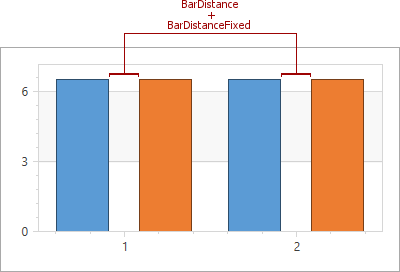20.2 (EAP/Beta)
20.1
19.2
19.1
18.2
18.1
17.2

# XYDiagram2D.BarDistance Property

Gets or sets the variable distance value (as a fraction of axis units) between two bars of different 2D Bar Series shown at the same argument point.

Namespace: DevExpress.Xpf.Charts

Assembly: DevExpress.Xpf.Charts.v20.1.dll

## Declaration

``public double BarDistance { get; set; }``
``Public Property BarDistance As Double``

## Property Value

Type Description
Double

A Double value that represents the distance between two neighboring bars as a fraction of axis units, where an axis unit is the distance between two major values on the axis.

## Remarks

The BarDistance property is introduced by the XYDiagram2D class, to synchronize it in all 2D Side-by-Side Bar series which are present in a chart.

The BarDistance property specifies the distance between two bars in a 2D Side-by-Side Bar Series. This property's value is given as a fraction, allowing the distance to be automatically resized when the size of the chart is changed. For instance, if the BarDistance property is set to 0.5, then the distance between the two bars will be always equal to half the range between the two major values on the axis.

To specify the distance between two bars in pixels, use the XYDiagram2D.BarDistanceFixed property. If both the BarDistance and XYDiagram2D.BarDistanceFixed properties are in effect at the same time, their values are summed as constant and variable distances.